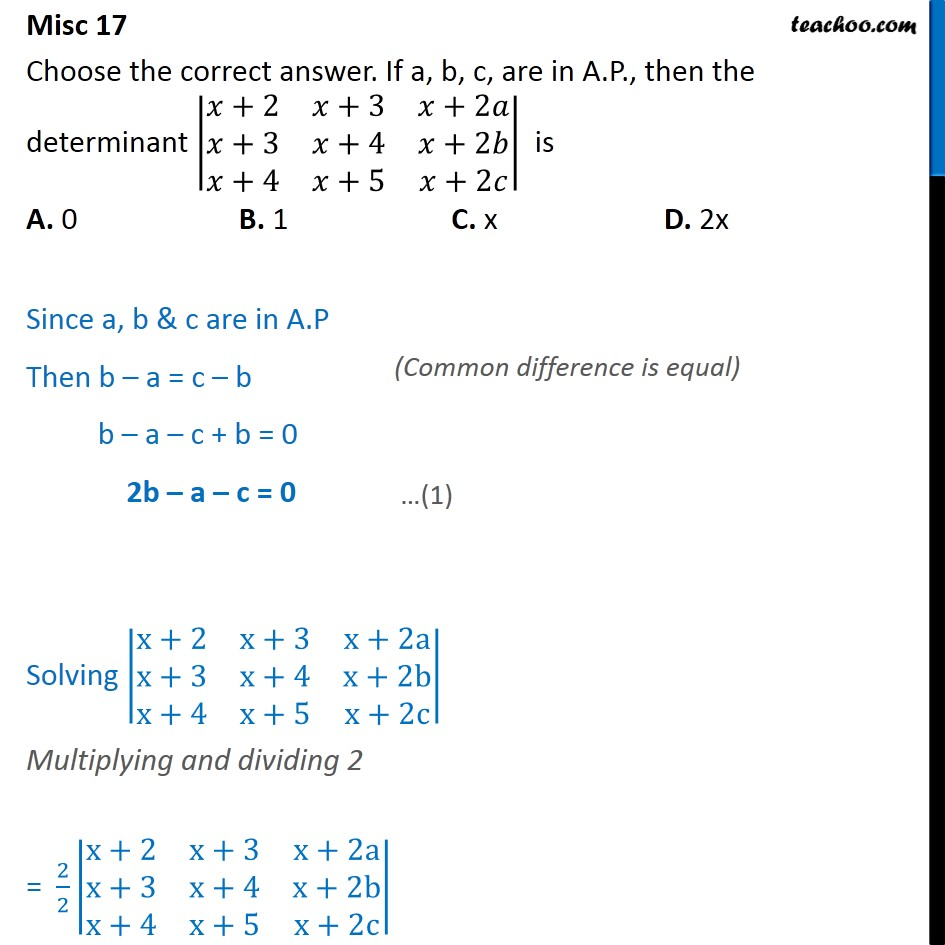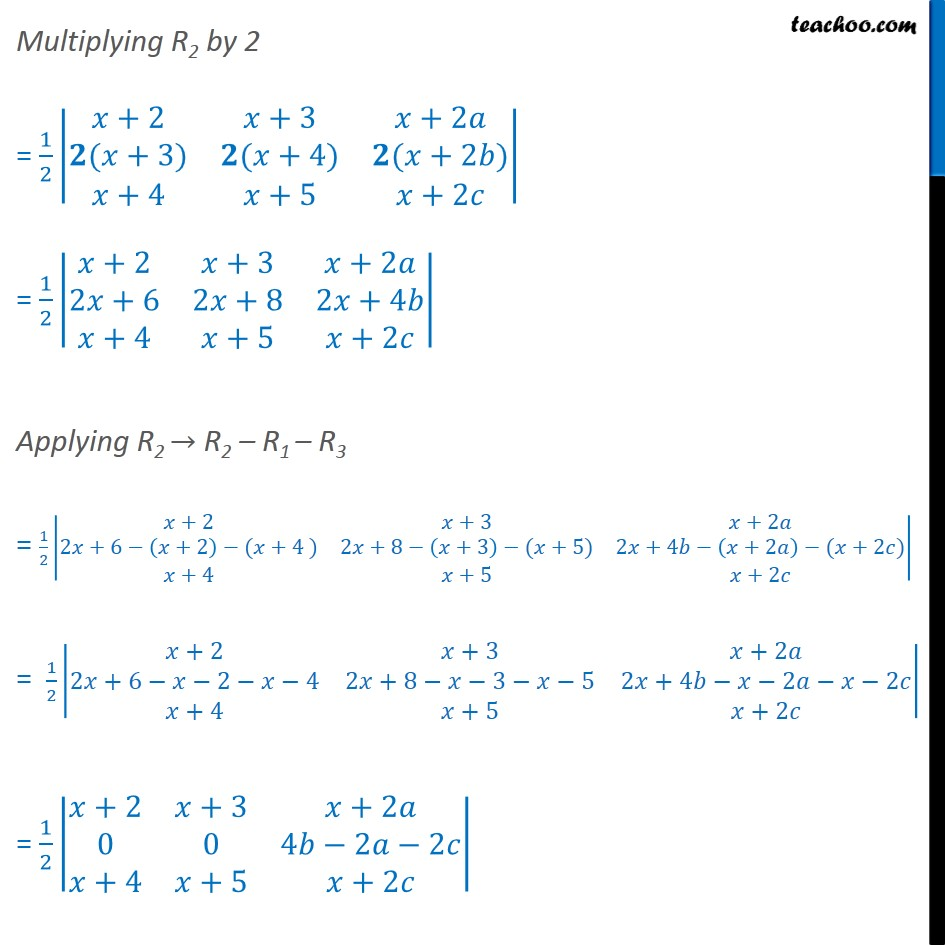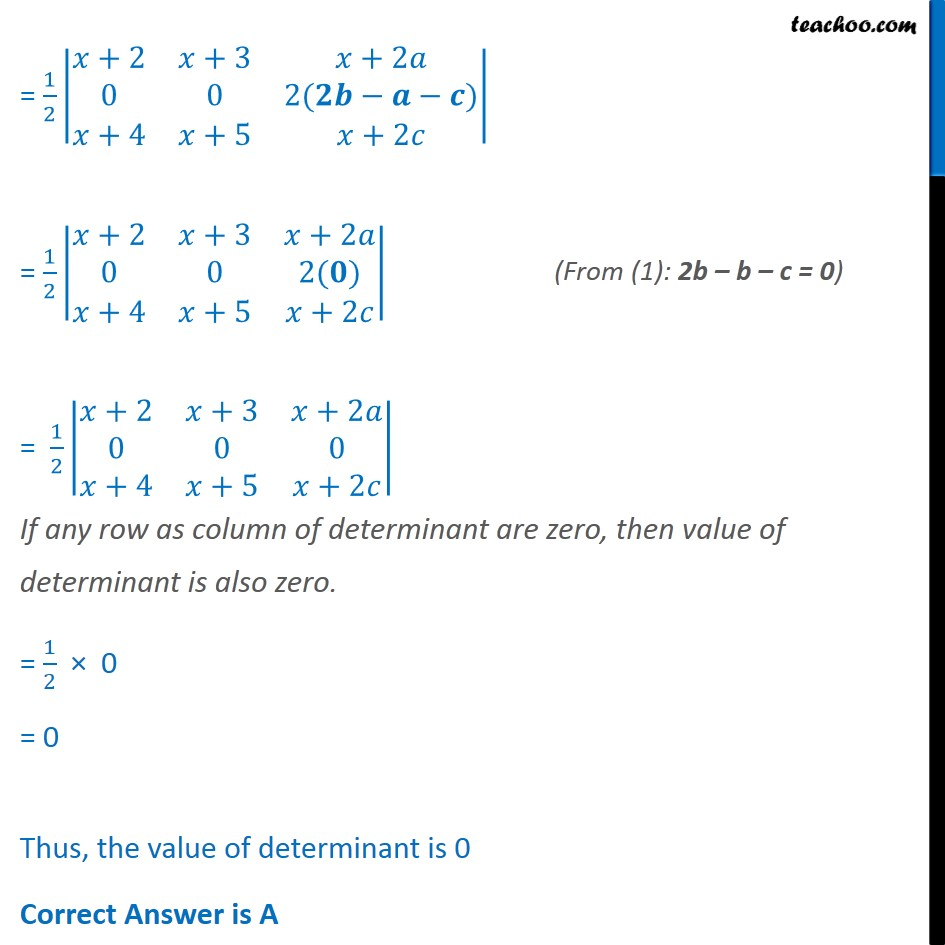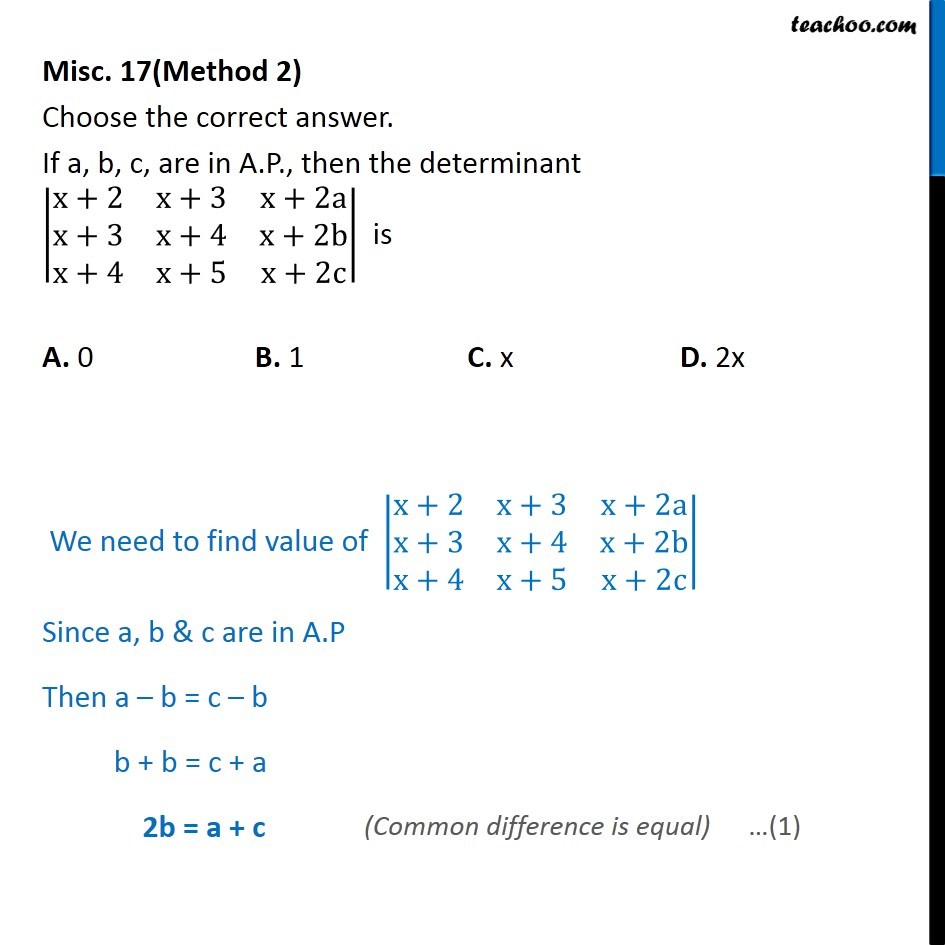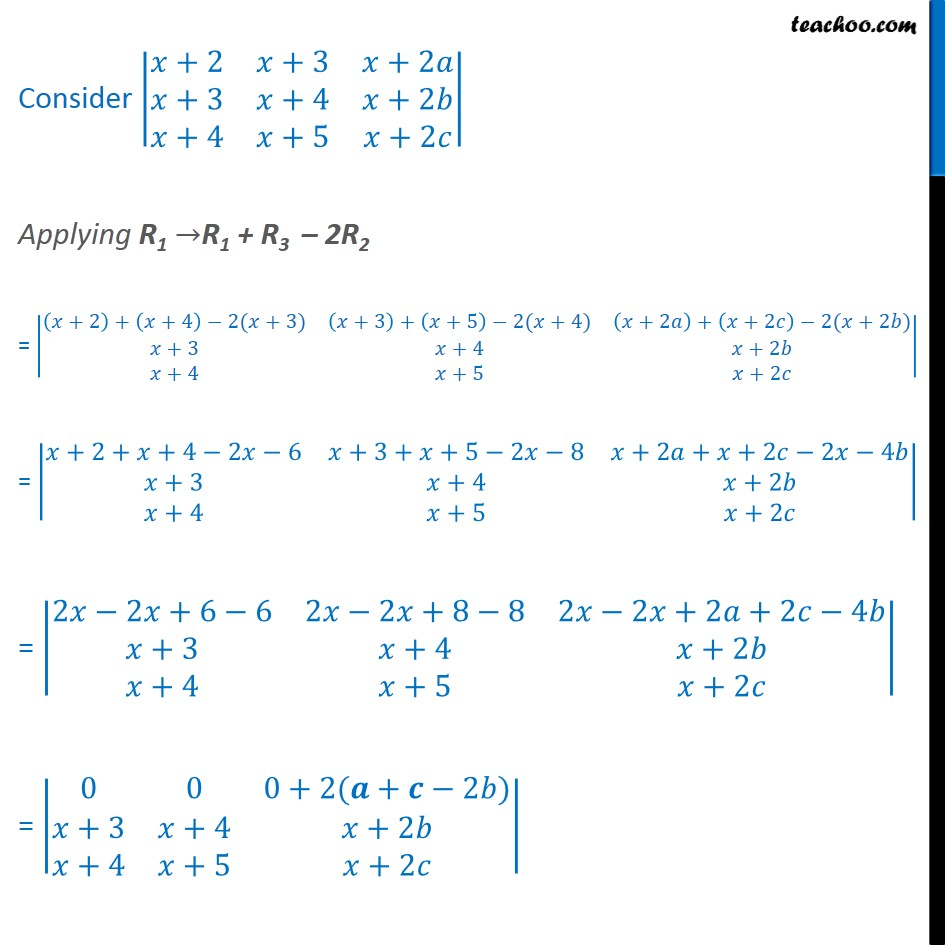1. Chapter 4 Class 12 Determinants
2. Serial order wise
3. Miscellaneous

Transcript

Misc 17 Choose the correct answer. If a, b, c, are in A.P., then the determinant +2 +3 +2 +3 +4 +2 +4 +5 +2 is A. 0 B. 1 C. x D. 2x Since a, b & c are in A.P Then b a = c b b a c + b = 0 2b a c = 0 Solving x+2 x+3 x+2a x+3 x+4 x+2b x+4 x+5 x+2c Multiplying and dividing 2 = 2 2 x+2 x+3 x+2a x+3 x+4 x+2b x+4 x+5 x+2c Multiplying R2 by 2 = 1 2 +2 +3 +2 ( +3) ( +4) ( +2 ) +4 +5 +2 = 1 2 +2 +3 +2 2 +6 2 +8 2 +4 +4 +5 +2 Applying R2 R2 R1 R3 = 1 2 +2 +3 +2 2 +6 +2 ( +4 ) 2 +8 +3 ( +5) 2 +4 +2 ( +2 ) +4 +5 +2 = 1 2 +2 +3 +2 2 +6 2 4 2 +8 3 5 2 +4 2 2 +4 +5 +2 = 1 2 +2 +3 +2 0 0 4 2 2 +4 +5 +2 = 1 2 +2 +3 +2 0 0 2( ) +4 +5 +2 = 1 2 +2 +3 +2 0 0 2( ) +4 +5 +2 = 1 2 +2 +3 +2 0 0 0 +4 +5 +2 If any row as column of determinant are zero, then value of determinant is also zero. = 1 2 0 = 0 Thus, the value of determinant is 0 Correct Answer is A Misc. 17(Method 2) Choose the correct answer. If a, b, c, are in A.P., then the determinant x+2 x+3 x+2a x+3 x+4 x+2b x+4 x+5 x+2c is A. 0 B. 1 C. x D. 2x We need to find value of x+2 x+3 x+2a x+3 x+4 x+2b x+4 x+5 x+2c Since a, b & c are in A.P Then a b = c b b + b = c + a 2b = a + c Consider +2 +3 +2 +3 +4 +2 +4 +5 +2 Applying R1 R1 + R3 2R2 = +2 + +4 2( +3) +3 + +5 2( +4) +2 + +2 2( +2 ) +3 +4 +2 +4 +5 +2 = +2+ +4 2 6 +3+ +5 2 8 +2 + +2 2 4 +3 +4 +2 +4 +5 +2 = 2 2 +6 6 2 2 +8 8 2 2 +2 +2 4 +3 +4 +2 +4 +5 +2 = 0 0 0+2( + 2 ) +3 +4 +2 +4 +5 +2 = 0 0 2( 2 ) +3 +4 +2 +4 +5 +2 = 0 0 0 +3 +4 +2 +4 +5 +2 If any row as column of determinant are zero, then value of determinant is also zero. = 0 Hence, value of determinant is 0 Correct Answer is A

Miscellaneous